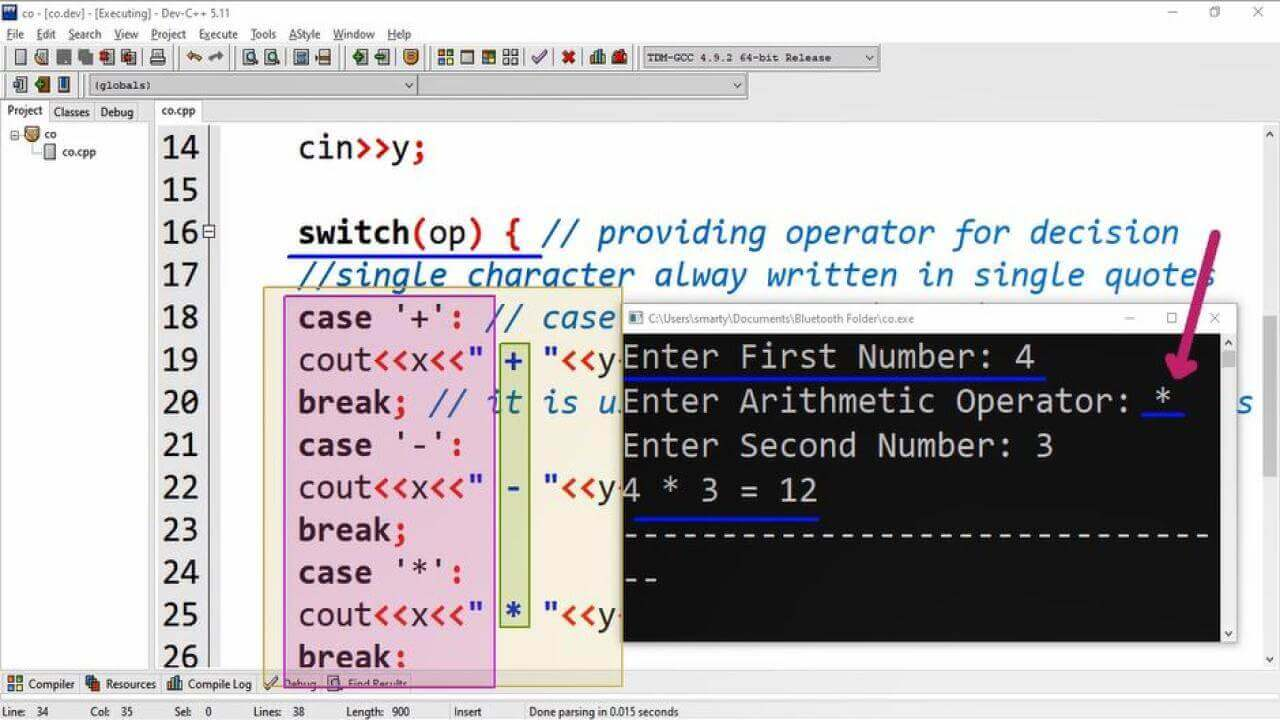# Simple Calculator Using Switch Statement In C++

## Source Code

#include <iostream>
using namespace std;
int main() {
int x,y; // variable declaration for taking input
char op; // variable for symbol / operator input
cout<<"Enter First Number: ";
cin>>x;

//Taking operator input
cout<<"Enter Arithmetic Operator: ";
cin>>op; // eg: +, -, *, /, %

cout<<"Enter Second Number: ";
cin>>y;

switch(op) { // providing operator for decision
//single character alway written in single quotes
case '+': // case ends with : (colon) symbol
cout<<x<<" + "<<y<<" = "<<x+y;
break; // it is used to skip next coming statements
case '-':
cout<<x<<" - "<<y<<" = "<<x-y;
break;
case '*':
cout<<x<<" * "<<y<<" = "<<x*y;
break;
case '/':
cout<<x<<" / "<<y<<" = "<<x/y;
break;
case '%':
cout<<x<<" % "<<y<<" = "<<x%y;
break;
default: // when no case will match/true
cout<<"Invalid Operator"<<endl;
}
return 0;
}

## OutputWorking:

In this program example we develop an arithmetic calculator. This can also said simple calculator because it will only perform the arithmetic operations such as multiplication, subtraction, addition, subtraction and modular of given numbers. This code starts from adding a standard library iostream that is mandatory for using basic input and output operations in program. Then we add standard namespace statement. If we do not want to add this namespace line in code then we can just use cin and cout statements by adding std:: class with entire cin and cout objects throughout the program. So the best practice is to use this line just after the include of header files.

Secondly in main function we declare three variables. These variables are declaed for the following purpose.

 Sr Name/identifier Type Purpose 1 x int Used to take first value input 2 y int Used to take second value input 3 op char This character variable is declared to take character input such as arithmetic operators.

After declaration of required variables we take input using cin object. The best practice to take input is to first prompt some message using cout object. Which will dispaly the required input description. Then we have to use cin object.

In last phase we provide the operator that is taken as input in op variable to switch statement. Switch satatement will match this provided operator with all written case statements in its body. If any case statement matches then the satements after the case statement will be excuted. All statements after case statement will be executed until the break statement comes.

Note if break statement is not written after case statements then all remaining statements will also executed including default statement.

At last if no case statement will true then the default statement will be executed. It is best practice to use defualt statement as the final statement in body of switch statement.

.